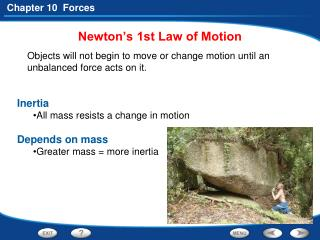DownloadDownload PresentationNewton’s 1st Law of Motion

# Newton’s 1st Law of Motion

Télécharger la présentation## Newton’s 1st Law of Motion

- - - - - - - - - - - - - - - - - - - - - - - - - - - E N D - - - - - - - - - - - - - - - - - - - - - - - - - - -
##### Presentation Transcript

1. Newton’s 1st Law of Motion • Objects will not begin to move or change motion until an unbalanced force acts on it. • Inertia • All mass resists a change in motion • Depends on mass • Greater mass = more inertia

2. Newton’s 2nd Law of Motion • Changes in force & mass • Acceleration increases • when force (push or pull) increases • when mass decreases

3. Newton’s 2nd Law of Motion • Determining Acceleration • Acceleration (motion) depends on how much force is used and the amount of mass. • acceleration = Force • mass

4. Newton’s 3rd Law of Motion • If one object exerts a force on another object, then the second object exerts a force back that is… • equal in strength and opposite in direction on the first object.

5. Momentum • “quantity of motion” depends on the mass and the velocity • Momentum (p) = mass (kg) x velocity (m/s)

6. - Conservation of Momentum • Momentum is conserved.. • when 2 objects collide • remains the same before and after the collision

7. A speedboat pulls a 55-kg water-skier. The skier to accelerates at 2.0 m/s2. Calculate the net force that causes this acceleration. What is the question asking for? What information have you been given? Mass of the water-skier (m) = 55 kg Acceleration of the water-skier (a) = 2.0 m/s2 Pg.392 Calculating Force

8. Practice Problem What is the net force on a 1,000-kg object accelerating at 3 m/s2? F = ma =1,000 kg x 3 m/s2= 3,000 N Pg.392 Calculating Force

9. Which has more momentum: a 3.0-kg sledgehammer swung at 1.5 m/s, or a 4.0-kg sledgehammer swung at 0.9 m/s? Read and Understand What information are you given? Mass of smaller sledgehammer = 3.0 kg Velocity of smaller sledgehammer = 1.5 m/s Mass of larger sledgehammer = 4.0 kg Velocity of larger sledgehammer = 0.9 m/s Calculating Momentum

10. Which has more momentum: a 3.0-kg sledgehammer swung at 1.5 m/s or a 4.0-kg sledgehammer swung at 0.9 m/s? What formula contains the given quantities and the unknown quantity? Momentum = Mass x Velocity p = mv Smaller sledgehammer = 3.0 km x 1.5 m/s = 4.5 kg•m/s Larger sledgehammer = 4.0 km x 0.9 m/s = 3.6 kg•m/s Calculating Momentum

11. Practice Problem A golf ball travels at 16 m/s, while a baseball moves at 7 m/s. The mass of the golf ball is 0.045 kg and the mass of the baseball is 0.14 kg. Which has greater momentum? Golf ball: 0.045 kg x 16 m/s = 0.72 kg•m/s Baseball: 0.14 kg x 7 m/s = 0.98 kg•m/s The baseball has greater momentum. Calculating Momentum

12. Practice Problem What is the momentum of a bird with a mass of 0.018 kg flying at 15 m/s? 0.27 kg•m/s (0.018 kg x 15 m/s = 0.27 kg•m/s) Calculating Momentum

13. Section 5:Rockets and Satellites • How does a rocket lift off the ground? • What keeps a satellite in orbit?

14. What Is a Satellite? • A projectile follows a curved path. The horizontal and vertical motions act independently.

15. What Is a Satellite? • The faster a projectile is thrown, the father it travels before it hits the ground. A projectile with enough velocity moves in a circular orbit.

16. What Is a Satellite? • Depending on their uses, artificial satellites orbit at different heights.

17. End of Section:Rockets and Satellites